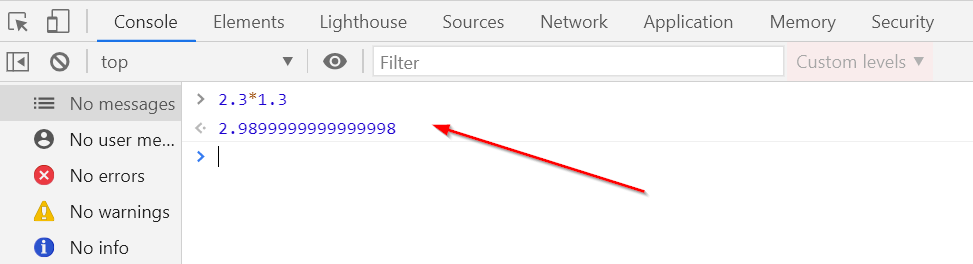Support » Plugin: Calculated Fields Form » Imprecise calculations issue

• Hi everyone!
Trying to calculate 21% tax of an amount. For example, of 22. Creating several fields:
Enter an amount (fieldname5)
Amount plus tax (fieldname5*1.21)
Amount minus tax (fieldname5*79)/100
Tax (fieldname5*0.21)
Calculate button

That is what happens:
Amount plus tax calculated as 26.619999999999997 although it’s 26.62 http://prntscr.com/uhyumq
If an amount is 10, Tax calculated as 2.0999999999999996 http://prntscr.com/uhyuhv if i set my formula to fieldname2-fieldname5
And same unpredictable mistakes from the plugin with other amounts, but most taxes calculated correctly

I have tried out different options of loading the forms, different encoding and change formulas, nothing helps. Not only one form but many of them guilty of this unpredictably

Can it be fixed please? Any idea how to do this?

Viewing 4 replies - 1 through 4 (of 4 total)
• Hello @derevco

Please, use the PREC operation in the equations. PREC(X,Y) rounds the number X with Y decimals.

So, the equations would be:

`PREC(fieldname5*1.21, 2)`

`PREC(fieldname5*79/100, 2)`

and

`PREC(fieldname5*0.21, 2)`

Best regards.

Hey, thanks @codepeople!
But why an unrounded result is imprecise? It kinda should be exact without rounding necessary

Hello @derevco

This issue is not caused by the plugin. This comes directly by the implementation of the Javascript language by the browsers. Please, look the screenshot below, it is not related with the plugin, I’ve evaluated the mathematical operation directly into the developers console of the browser: